NCERT Solutions for Class 9 Maths Chapter 11 Constructions Ex 11.1 are part of NCERT Solutions for Class 9 Maths. Here we have given NCERT Solutions for Class 9 Maths Chapter 11 Constructions Ex 11.1.

## NCERT Solutions for Class 9 Maths Chapter 11 Constructions Ex 11.1

Ex 11.1 Class 9 Maths Question 1.
Construct an angle of 90° at the initial point of a given ray and justify the construction.
Solution:
Steprf of Construction:
Step I : Draw $$\overline { AB }$$.
Step II : Taking O as centre and having a suitable radius, draw a semicircle, which cuts $$\overline { OA }$$ at B.
Step III : Keeping the radius same, divide the semicircle into three equal parts such that $$\breve { BC } =\quad \breve { CD } \quad =\quad \breve { DE }$$ .
Step IV : Draw $$\overline { OC }$$ and $$\overline { OD }$$.
Step V : Draw $$\overline { OF }$$, the bisector of ∠COD.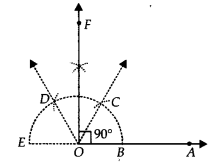Thus, ∠AOF = 90°
Justification:
∵ O is the centre of the semicircle and it is divided into 3 equal parts.
∴ $$\breve { BC } =\quad \breve { CD } \quad =\quad \breve { DE }$$
⇒ ∠BOC = ∠COD = ∠DOE [Equal chords subtend equal angles at the centre]
And, ∠BOC + ∠COD + ∠DOE = 180°
⇒ ∠BOC + ∠BOC + ∠BOC = 180°
⇒ 3∠BOC = 180°
⇒ ∠BOC = 60°
Similarly, ∠COD = 60° and ∠DOE = 60°
∵ $$\overline { OF }$$ is the bisector of ∠COD
∴ ∠COF = $$\frac { 1 }{ 2 }$$ ∠COD = $$\frac { 1 }{ 2 }$$ (60°) = 30°
Now, ∠BOC + ∠COF = 60° + 30°
⇒ ∠BOF = 90° or ∠AOF = 90°

Ex 11.1 Class 9 Maths Question 2.
Construct an angle of 45° at the initial point of a given ray and justify the construction.
Solution:
Steps of Construction:
Stept I : Draw $$\overline { OA }$$.
Step II : Taking O as centre and with a suitable radius, draw a semicircle such that it intersects $$\overline { OA }$$. at B.
Step III : Taking B as centre and keeping the same radius, cut the semicircle at C. Now, taking C as centre and keeping the same radius, cut the semicircle at D and similarly, cut at E, such that $$\breve { BC } =\quad \breve { CD } \quad =\quad \breve { DE }$$
Step IV : Draw $$\overline { OC }$$ and $$\overline { OD }$$.
Step V : Draw $$\overline { OF }$$, the angle bisector of ∠BOC.
Step VI : Draw $$\overline { OG }$$, the ajngle bisector of ∠FOC.Thus, ∠BOG = 45° or ∠AOG = 45°
Justification:
∵ $$\breve { BC } =\quad \breve { CD } \quad =\quad \breve { DE }$$
∴ ∠BOC = ∠COD = ∠DOE [Equal chords subtend equal angles at the centre]
Since, ∠BOC + ∠COD + ∠DOE = 180°
⇒ ∠BOC = 60°
∵ $$\overline { OF }$$ is the bisector of ∠BOC.
∴ ∠COF = $$\frac { 1 }{ 2 }$$ ∠BOC = $$\frac { 1 }{ 2 }$$(60°) = 30° …(1)
Also, $$\overline { OG }$$ is the bisector of ∠COF.
∠FOG = $$\frac { 1 }{ 2 }$$∠COF = $$\frac { 1 }{ 2 }$$(30°) = 15° …(2)
Adding (1) and (2), we get
∠COF + ∠FOG = 30° + 15° = 45°
⇒ ∠BOF + ∠FOG = 45° [∵ ∠COF = ∠BOF]
⇒ ∠BOG = 45°

Ex 11.1 Class 9 Maths Question 3.
Construct the angles of the following measurements
(i) 30°
(ii) 22 $$\frac { 1 }{ 2 } \circ$$
(iii) 15°
Solution:
(i) Angle of 30°
Steps of Construction:
Step I : Draw $$\overline { OA }$$.
Step II : With O as centre and having a suitable radius, draw an arc cutting $$\overline { OA }$$ at B.
Step III : With centre at B and keeping the same radius as above, draw an arc to cut the previous arc at C.
Step IV : Join $$\overline { OC }$$ which gives ∠BOC = 60°.
Step V : Draw $$\overline { OD }$$, bisector of ∠BOC, such that ∠BOD = $$\frac { 1 }{ 2 }$$∠BOC = $$\frac { 1 }{ 2 }$$(60°) = 30°Thus, ∠BOD = 30° or ∠AOD = 30°

(ii) Angle of 22 $$\frac { 1 }{ 2 } \circ$$
Steps of Construction:
Step I : Draw $$\overline { OA }$$.
Step II : Construct ∠AOB = 90°
Step III : Draw $$\overline { OC }$$, the bisector of ∠AOB, such that
∠AOC = $$\frac { 1 }{ 2 }$$∠AOB = $$\frac { 1 }{ 2 }$$(90°) = 45°
Step IV : Now, draw OD, the bisector of ∠AOC, such that
∠AOD = $$\frac { 1 }{ 2 }$$∠AOC = $$\frac { 1 }{ 2 }$$(45°) = 22 $$\frac { 1 }{ 2 } \circ$$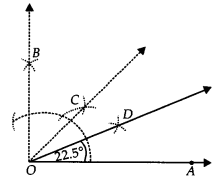Thus, ∠AOD = 22 $$\frac { 1 }{ 2 } \circ$$

(iii) Angle of 15°
Steps of Construction:
Step I : Draw $$\overline { OA }$$.
Step II : Construct ∠AOB = 60°.
Step III : Draw OC, the bisector of ∠AOB, such that
∠AOC = $$\frac { 1 }{ 2 }$$∠AOB = $$\frac { 1 }{ 2 }$$(60°) = 30°
i.e., ∠AOC = 30°
Step IV : Draw OD, the bisector of ∠AOC such that
∠AOD = $$\frac { 1 }{ 2 }$$∠AOC = $$\frac { 1 }{ 2 }$$(30°) = 15°Thus, ∠AOD = 15°

Ex 11.1 Class 9 Maths Question 4.
Construct the following angles and verify by measuring them by a protractor
(i) 75°
(ii) 105°
(iii) 135°
Solution:
Step I : Draw $$\overline { OA }$$.
Step II : With O as centre and having a suitable radius, draw an arc which cuts $$\overline { OA }$$ at B.
Step III : With centre B and keeping the same radius, mark a point C on the previous arc.
Step IV : With centre C and having the same radius, mark another point D on the arc of step II.
Step V : Join $$\overline { OC }$$ and $$\overline { OD }$$, which gives ∠COD = 60° = ∠BOC.
Step VI : Draw $$\overline { OP }$$, the bisector of ∠COD, such that
∠COP = $$\frac { 1 }{ 2 }$$∠COD = $$\frac { 1 }{ 2 }$$(60°) = 30°.
Step VII: Draw $$\overline { OQ }$$, the bisector of ∠COP, such that
∠COQ = $$\frac { 1 }{ 2 }$$∠COP = $$\frac { 1 }{ 2 }$$(30°) = 15°.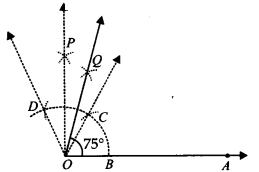Thus, ∠BOQ = 60° + 15° = 75°∠AOQ = 75°

(ii) Steps of Construction:
Step I : Draw $$\overline { OA }$$.
Step II : With centre O and having a suitable radius, draw an arc which cuts $$\overline { OA }$$ at B.
Step III : With centre B and keeping the same radius, mark a point C on the previous arc.
Step IV : With centre C and having the same radius, mark another point D on the arc drawn in step II.
Step V : Draw OP, the bisector of CD which cuts CD at E such that ∠BOP = 90°.
Step VI : Draw $$\overline { OQ }$$, the bisector of $$\breve { BC }$$ such that ∠POQ = 15°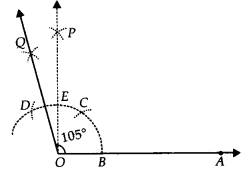Thus, ∠AOQ = 90° + 15° = 105°

(iii) Steps of Construction:
Step I : Draw $$\overline { OP }$$.
Step II : With centre O and having a suitable radius, draw an arc which cuts $$\overline { OP }$$ at A
Step III : Keeping the same radius and starting from A, mark points Q, R and S on the arc of step II such that $$\breve { AQ } =\quad \breve { QR } \quad =\quad \breve { RS }$$ .
StepIV :Draw $$\overline { OL }$$, thebisector of $$\breve { RS }$$ which cuts the arc $$\breve { RS }$$ at T.
Step V : Draw $$\overline { OM }$$, the bisector of $$\breve { RT }$$.Thus, ∠POQ = 135°

Ex 11.1 Class 9 Maths Question 5.
Construct an equilateral triangle, given its side and justify the construction.
Solution:
pt us construct an equilateral triangle, each of whose side = 3 cm(say).
Steps of Construction:
Step I : Draw $$\overline { OA }$$.
Step II : Taking O as centre and radius equal to 3 cm, draw an arc to cut $$\overline { OA }$$ at B such that OB = 3 cm
Step III : Taking B as centre and radius equal to OB, draw an arc to intersect the previous arc at C.
Step IV : Join OC and BC.Thus, ∆OBC is the required equilateral triangle.

Justification:
∵ The arcs $$\breve { OC }$$ and $$\breve { BC }$$ are drawn with the same radius.
∴ $$\breve { OC }$$ = $$\breve { BC }$$
⇒ OC = BC [Chords corresponding to equal arcs are equal]
∵ OC = OB = BC
∴ OBC is an equilateral triangle.

### NCERT Solutions for Class 9 Maths Chapter 11 Constructions (Hindi Medium) Ex 11.1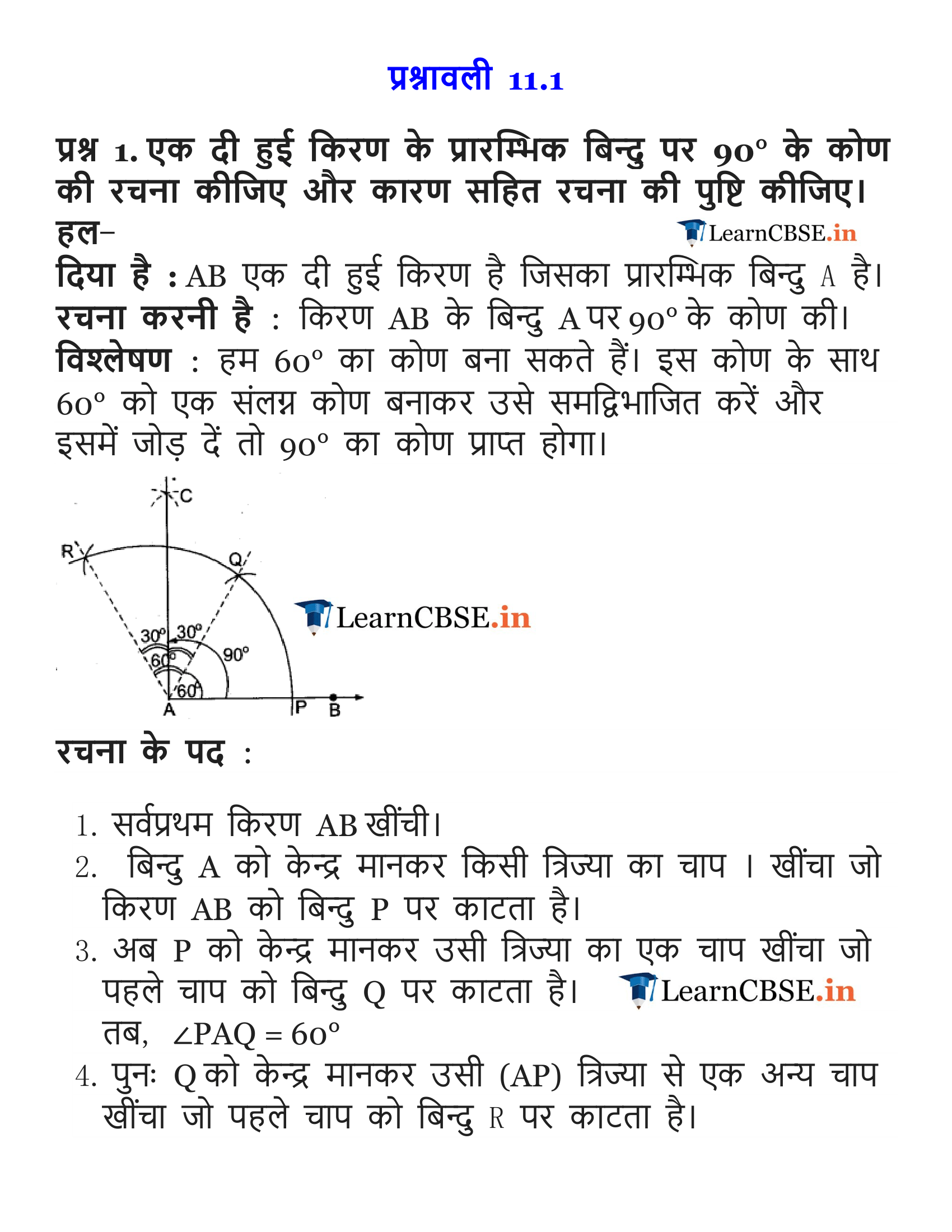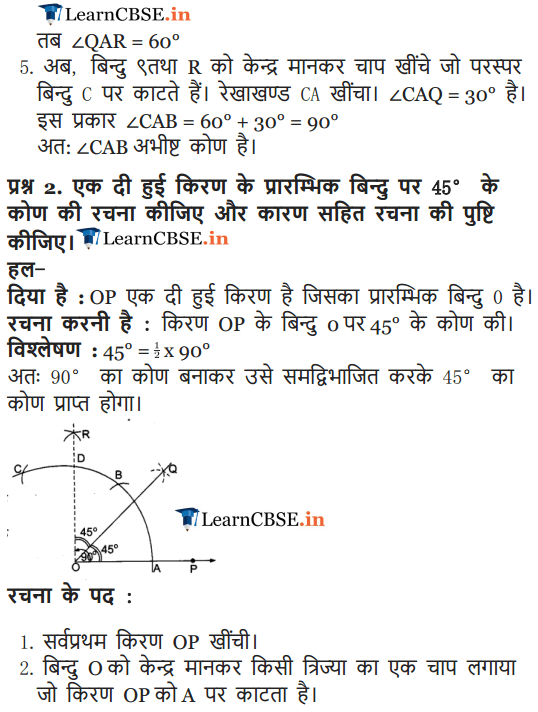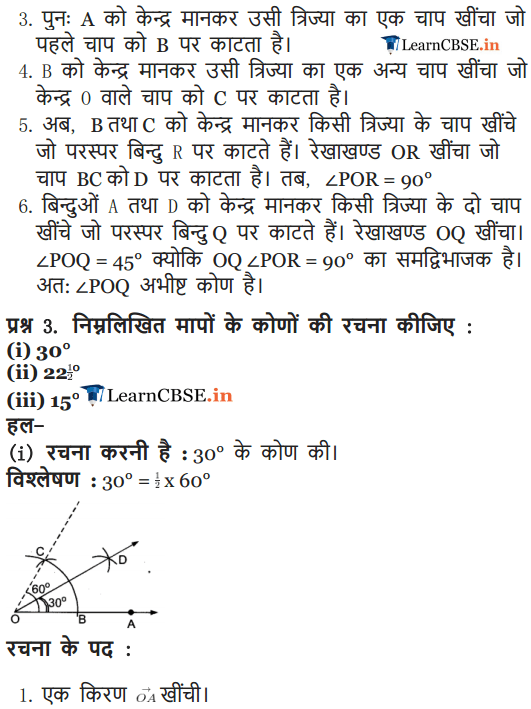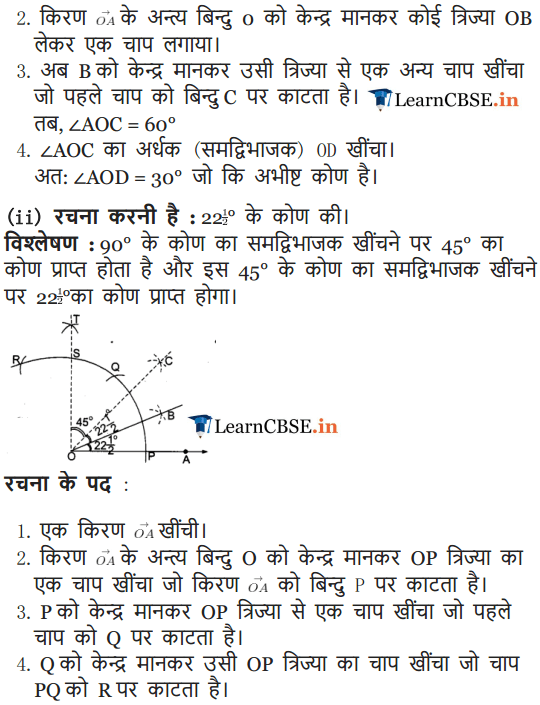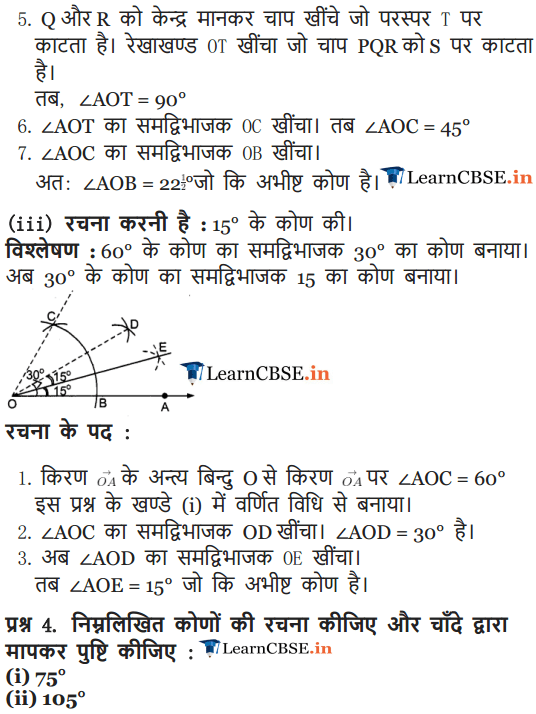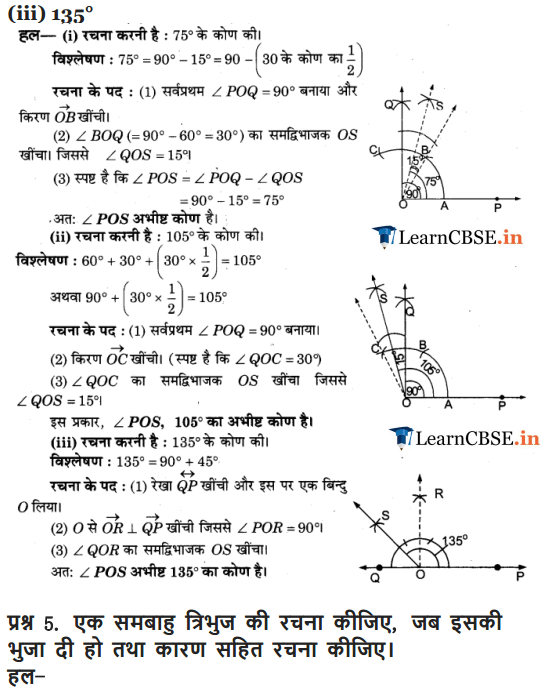### NCERT Solutions for Class 9 Maths Chapter 11 Constructions Ex 11.2

Ex 11.2 Class 9 Maths Question 1.
Construct a ∆ ABC in which BC = 7 cm, ∠B = 75° and AB + AC = 13 cm.
Solution:
Steps of Construction:
Step I : Draw $$\overline { BX }$$.
Step II : Along $$\overline { BX }$$, cut off a line segment BC = 7 cm.
Step III : At B, construct ∠CBY = 75°
Step IV : From $$\overline { BY }$$, cut off BD = 13 cm (= AB + AC)
Step V : Join DC.
Step VI : Draw a perpendicular bisector of CD which meets BD at A.
Step VII: Join AC.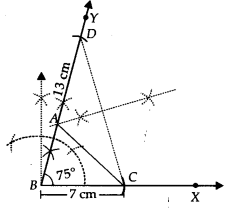Thus, ∆ABC is the required triangle.

Ex 11.2 Class 9 Maths Question 2.
Construct a ABC in which BC = 8 cm, ∠B = 45° and AB – AC = 35 cm.
Solution:
Steps of Construction:
Step I : Draw $$\overline { BX }$$.
Step II : Along $$\overline { BX }$$, cut off a line segment BC = 8 cm.
Step III : At B, construct ∠CBY = 45°
Step IV : From $$\overline { BX }$$, cut off BD = 3.5 cm (= AB – AC)
Step V : Join DC.
Step VI : Draw PQ, perpendicular bisector of DC, which intersects $$\overline { BY }$$ at A.
Step VII: Join AC.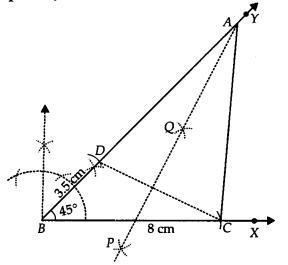Thus, ∆ABC is the required triangle.

Ex 11.2 Class 9 Maths Question 3.
Construct a ∆ ABC in which QR = 6 cm, ∠Q = 60° and PR – PQ = 2 cm.
Solution:
Steps of Construction:
Step I : Draw $$\overline { QX }$$.
Step II : Along $$\overline { QX }$$, cut off a line segment QR = 6 cm.
Step III : Construct a line YQY’ such that ∠RQY = 60°.
Step IV : Cut off QS = 2 cm (= PR – PQ) on QY’.
Step V : Join SR.
Step VI : Draw MN, perpendicular bisector of SR, which intersects QY at P.
Step VII: Join PR.Thus, ∆PQR is the required triangle.

Ex 11.2 Class 9 Maths Question 4.
Construct a ∆ XYZ in which ∠Y = 30°, ∠Y = 90° and XY + YZ + ZX = 11 cm.
Solution:
Steps of Construction:
Step I : Draw a line segment AB = 11 cm = (XY+YZ + ZX)
Step II : Construct ∠BAP = 30°
Step III : Construct ∠ABQ = 90°
Step IV : Draw AR, the bisector of ∠BAP.
Step V : Draw BS, the bisector of ∠ABQ. Let AR and BS intersect at X.
Step VI : Draw perpendicular bisector of $$\overline { AX }$$, which intersects AB at Y.
Step VII: Draw perpendicular bisector of $$\overline { XB }$$, which intersects AB at Z.
Step VIII: Join XY and XZ.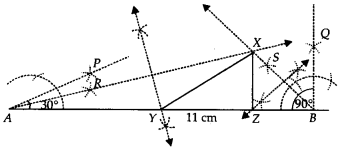Thus, ∆XYZ is the required triangle.

Ex 11.2 Class 9 Maths Question 5.
Construct a right triangle whose base is 12 cm and sum of its hypotenuse and other side is 18 cm.
Solution:
Steps of Construction:
Step I : Draw BC = 12 cm.
Step II : At B, construct ∠CBY = 90°.
Step III : Along $$\overline { BY }$$, cut off a line segment BX = 18 cm.
Step IV : Join CX.
Step V : Draw PQ, perpendicular bisector of CX, which meets BX at A.
Step VI : Join AC.Thus, ∆ABC is the required triangle.

We hope the NCERT Solutions for Class 9 Maths Chapter 11 Constructions Ex 11.1 help you. If you have any query regarding NCERT Solutions for Class 9 Maths Chapter 11 Constructions Ex 11.1, drop a comment below and we will get back to you at the earliest.